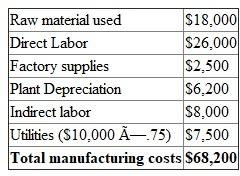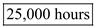## Quiz 13 : Cost Accounting and ReportingLooking for Accounting Homework Help?# Quiz 13 : Cost Accounting and Reporting

Manufacturing costs are those costs that are incurred to manufacture a product. Manufacturing costs include direct material, direct labor and manufacturing overheads. It means all product costs are included in the manufacturing cost. Manufacturing cost does not include administrative and marketing costs. The total manufacturing costs of the company for May is computed below:The total manufacturing costs of the company is \$68,200. Factory supplies costs, plant depreciation costs, indirect labor and utilities (75% relates to the factory) are manufacturing overheads and hence included in the total manufacturing costs. Only 75% of the utilities are related to the factory and therefore only 75% of the utilities cost is included in manufacturing costs. Sales expense, advertising costs and administrative expense are nonmanufacturing costs and hence not included in the manufacturing costs.

Calculate B's predetermined overhead rate: Predetermined overhead is the rater per unit of activity used to apply the manufacturing overhead to the work in process. It is calculated by dividing the estimated overhead costs in acivitity cost pool with the estimated number of activity units. Use the following formula to calculate the predetermined overhead rate:Therefore, predetermined overhead rate is \$2.5. Calculate cost per unit: Cost per unit is the total cost required to make a unit of product. It is calculated by dividing the sum of materials, labor and overheads with the number of units produced. Use the following formula to determine the cost per unit:Hence, the cost per unit is \$2.76.

Direct Labor: Direct Labor means that an effort provided by workers and directly involved in the manufacturing of a product. Calculate direct labor hours did by T Company incur during August: T Company applies manufacturing overhead to production at the rate of \$4 per direct labor hour. Actual manufacturing overhead incurred for August amounted to \$88,000 Under applied overhead is \$12,000 Assume that the amount of direct labor hours be' a'Therefore, the amount of direct labor hours was.